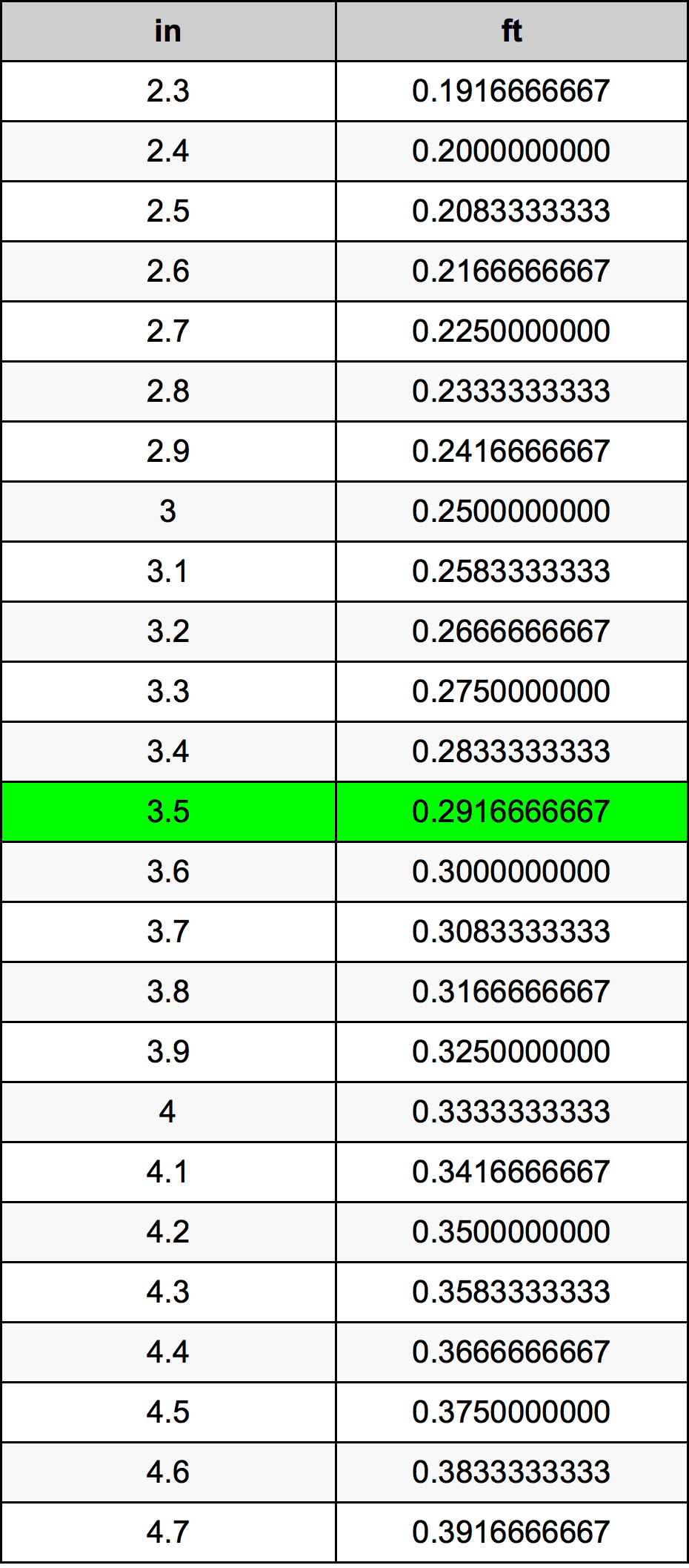Inches To Feet

# 3.5 in to ft3.5 Inches to Feet

in
=
ft

## How to convert 3.5 inches to feet?

 3.5 in * 0.0833333333 ft = 0.2916666667 ft 1 in
A common question is How many inch in 3.5 foot? And the answer is 42.0 in in 3.5 ft. Likewise the question how many foot in 3.5 inch has the answer of 0.2916666667 ft in 3.5 in.

## How much are 3.5 inches in feet?

3.5 inches equal 0.2916666667 feet (3.5in = 0.2916666667ft). Converting 3.5 in to ft is easy. Simply use our calculator above, or apply the formula to change the length 3.5 in to ft.

## Convert 3.5 in to common lengths

UnitUnit of length
Nanometer88900000.0 nm
Micrometer88900.0 µm
Millimeter88.9 mm
Centimeter8.89 cm
Inch3.5 in
Foot0.2916666667 ft
Yard0.0972222222 yd
Meter0.0889 m
Kilometer8.89e-05 km
Mile5.52399e-05 mi
Nautical mile4.80022e-05 nmi

## What is 3.5 inches in ft?

To convert 3.5 in to ft multiply the length in inches by 0.0833333333. The 3.5 in in ft formula is [ft] = 3.5 * 0.0833333333. Thus, for 3.5 inches in foot we get 0.2916666667 ft.

## 3.5 Inch Conversion Table## Alternative spelling

3.5 Inch to Feet, 3.5 Inch in Feet, 3.5 in to Foot, 3.5 in in Foot, 3.5 Inch to ft, 3.5 Inch in ft, 3.5 in to ft, 3.5 in in ft, 3.5 Inches to ft, 3.5 Inches in ft, 3.5 Inch to Foot, 3.5 Inch in Foot, 3.5 Inches to Foot, 3.5 Inches in Foot